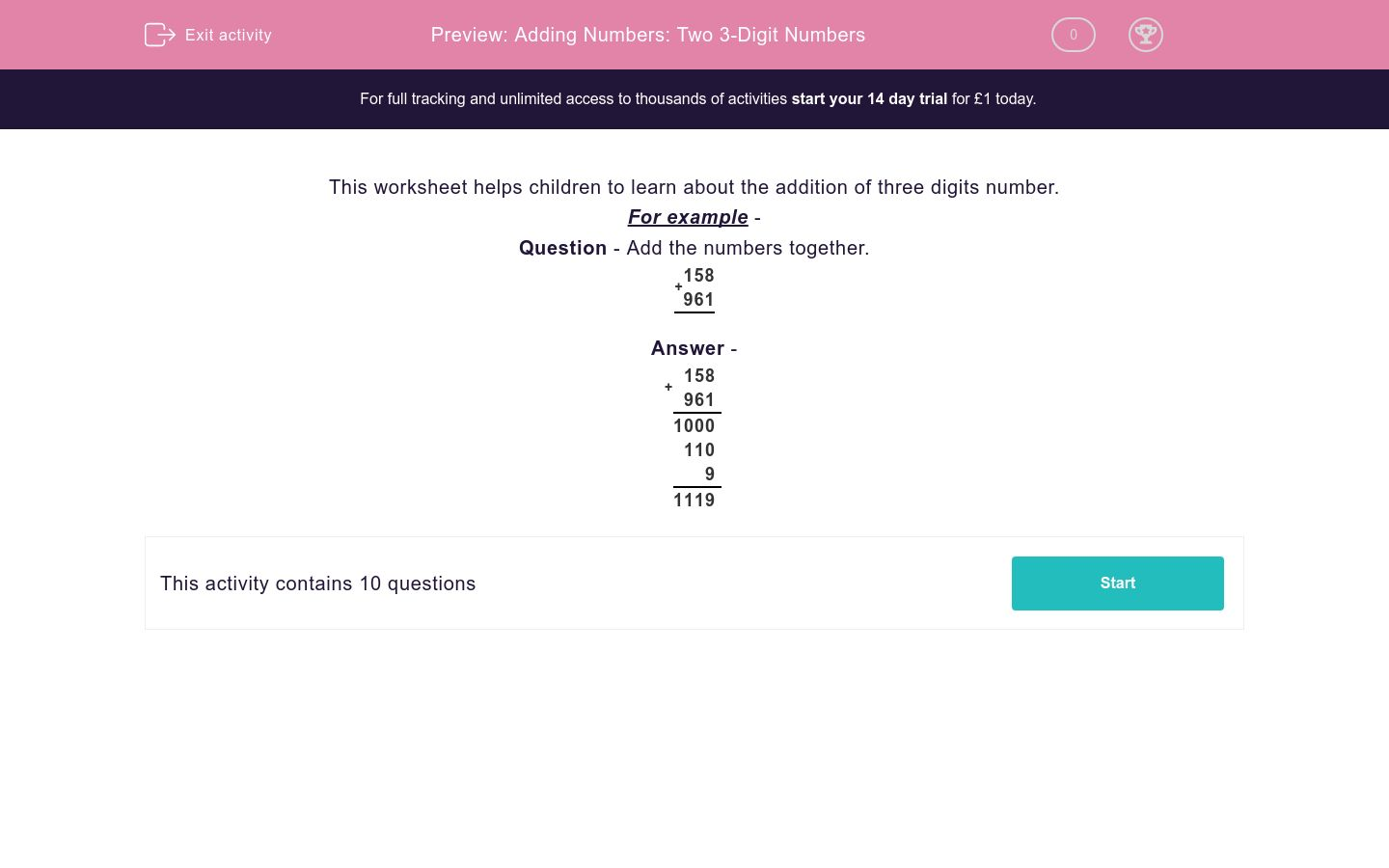# Adding Numbers: Two 3-Digit Numbers

In this worksheet, students add together two numbers - both of which have three digits. They use a formal written/column method.Key stage:  KS 2

Curriculum topic:   Maths and Numerical Reasoning

Curriculum subtopic:   Mixed Problems

Difficulty level:#### Worksheet Overview

This worksheet helps children to learn about the addition of three digits number.

For example -

Question - Add the numbers together.

 + 1 5 8 9 6 1